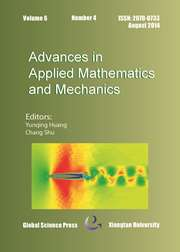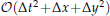Home
Hostname: page-component-6c8bd87754-lkb8j Total loading time: 0.191 Render date: 2022-01-21T05:20:38.579Z Has data issue: true Feature Flags: { "shouldUseShareProductTool": true, "shouldUseHypothesis": true, "isUnsiloEnabled": true, "metricsAbstractViews": false, "figures": true, "newCiteModal": false, "newCitedByModal": true, "newEcommerce": true, "newUsageEvents": true }Advances in Applied Mathematics and Mechanics

Multi-Symplectic Method for the Zakharov-Kuznetsov Equation

Published online by Cambridge University Press:  09 January 2015

*
*Email:sunjq123@qq.com(J. Q. Sun)

Abstract

A new scheme for the Zakharov-Kuznetsov (ZK) equation with the accuracy order ofis proposed. The multi-symplectic conservation property of the new scheme is proved. The backward error analysis of the new multi-symplectic scheme is also implemented. The solitary wave evolution behaviors of the Zakharov-Kunetsov equation is investigated by the new multi-symplectic scheme. The accuracy of the scheme is analyzed.

Type
Research Article
Information
Advances in Applied Mathematics and Mechanics , February 2015 , pp. 58 - 73

Access options

Get access to the full version of this content by using one of the access options below. (Log in options will check for institutional or personal access. Content may require purchase if you do not have access.)

References

Ablowitz, M. J. and Schober, C. M., Hamiltonian integrators for the nonlinear Schrödinger equation, Int. J. Modern Phys. C, 5 (1994), pp. 397401.CrossRefGoogle Scholar
Bridges, T. J., Transverse instability of solitary-wave states of the water-wave problem, J. Fluid Mech., 439 (2001), pp. 255278.CrossRefGoogle Scholar
Bridges, T. J., Universal geometric condition for the transverse instability of solitary waves, Phys. Rev. Lett., 84 (2000), pp. 26142617.CrossRefGoogle ScholarPubMed
Bridges, T. J., A geometric formulation of the conservation of wave action and its implications for signature and the classification of instabilities, Proc. Royal. Soc. London A, 453 (1997), pp. 13651395.CrossRefGoogle Scholar
Bridges, T. J. and Reich, S., Multi-symplectic spectral discretizations for the Zakharov-Kuznetsov and shallow-water equations, Phys. D, 152 (2001), pp. 491504.CrossRefGoogle Scholar
Bridges, T. J. and Reich, S., Numerical methods for Hamiltonian PDEs, J. Phys. A Math. Gen., 39 (2006), pp. 52875320.CrossRefGoogle Scholar
Bridges, T. J. and Reich, S., Multi-symplectic integrators: numerical schemes for Hamiltonian PDEs that conserve symplecticity, Phys. Lett. A, 284 (2001), pp. 184193.CrossRefGoogle Scholar
Blanes, S. and Moan, P. C., Splitting methods for the time-dependent Schrödinger equation, Phys. Lett. A, 265 (2000), pp. 3542.CrossRefGoogle Scholar
Chen, J. B., Multisymplectic geometry, local conservation laws and a multisymplectic integrator for the Zakharov-Kuznetsov equation, Lett. Math. Phys., 63 (2003), pp. 115124.CrossRefGoogle Scholar
Chen, J. B., A multisymplectic integrator for the periodic nonlinear Schrodinger equation, Appl. Math. Comput., 170 (2005), pp. 13941417.Google Scholar
Feng, K., Collected Works of Feng Kang II, National Defence Industry Press, Beijing, 1995.Google Scholar
Frank, J., Moore, B. and Reich, S., Linear PDEs and numerical mehods that preserve a multi-symplectic conservation law, SIAM J. Sci. Comput., 28 (2006), pp. 260277.CrossRefGoogle Scholar
Infeld, E., Self-focusing of nonlinear ion acoustic waves and solitons in magnetized plasmas, J. Plasma Phys., 33 (1985), pp. 171182.CrossRefGoogle Scholar
Infeld, E., Skorupski, A. A. and Senatorski, A., Dynamics of waves and multidimensional solitons of the Zakharov-Kuznetsov equation, J. Plasma Phys., 64 (2000), pp. 397409.CrossRefGoogle Scholar
Iwasaki, S., Toh, and Kawahara, T., Cylindrical qusi-solitons to the Zakharov-Kuznetsov equation, Phys. D, 43 (1990), pp. 293303.CrossRefGoogle Scholar
Kuznetsov, E. A., Rubenchik, A. M. and Zakharov, V. E., Soliton stability in plasmas and hydrodynamics, Phys. Rep., 142 (1986), pp. 103165.CrossRefGoogle Scholar
Kong, L. H., Hong, J. L. and Zhang, J. J., Splitting multisymplectic integrators for Maxwell’s equations, J. Comput. Phys., 229 (2010), pp. 42594278.CrossRefGoogle Scholar
Aydin, A. and Karasozen, B., Multisymplectic box scheme for the complex modified Kortewegde Vries equation, J. Math. Phys., 51 (2010), 083511.CrossRefGoogle Scholar
Moore, B. and Reich, S., Backward error analysis for multi-symplectic integration methods, Nu-merische Mathematik, 95 (2003), pp. 625652.CrossRefGoogle Scholar
Marsden, J. E., Patrick, G. P. and Shkoller, S., Multi-symplectic geometry, variational integrators, and nonlinear PDEs, Commun. Math. Phys., 199 (1999), pp. 351395.CrossRefGoogle Scholar
Nozaki, K., Vortex solitons of drift waves and anomalous diffusion, Phys. Rev. Lett., 46 (1981), pp. 184187.CrossRefGoogle Scholar
Petviashvili, V. I., Red spot of Jupiter and the drift soliton in a plasma, JETP Lett., 32 (1980), pp. 619622.Google Scholar
Reich, S., Backward error analysis for numerical integrators, SIAM J. Numer. Anal., 36 (1999), 1549.CrossRefGoogle Scholar
Sun, J. Q. and Qin, M. Z., Multi-symplectic methods for the coupled 1D nonlinear Schrödinger system, Comput. Phys. Commun., 155 (2003), pp. 221235.CrossRefGoogle Scholar
Wang, Y. S., Wang, B. and Chen, X., Multi-symplectic Euler-box scheme for the KdV equation, China Phys. Lett., 24 (2007), pp. 312314.Google Scholar
Zakharov, V. E. and Kuznetsov, E. A., Three-dimensional solitons, Soviet. Phys., 39 (1974), pp. 285286.Google Scholar
4
Cited by

Send article to Kindle

Note you can select to send to either the @free.kindle.com or @kindle.com variations. ‘@free.kindle.com’ emails are free but can only be sent to your device when it is connected to wi-fi. ‘@kindle.com’ emails can be delivered even when you are not connected to wi-fi, but note that service fees apply.

Find out more about the Kindle Personal Document Service.

Multi-Symplectic Method for the Zakharov-Kuznetsov Equation
Available formats
×

Send article to Dropbox

To send this article to your Dropbox account, please select one or more formats and confirm that you agree to abide by our usage policies. If this is the first time you use this feature, you will be asked to authorise Cambridge Core to connect with your <service> account. Find out more about sending content to Dropbox.

Multi-Symplectic Method for the Zakharov-Kuznetsov Equation
Available formats
×

To send this article to your Google Drive account, please select one or more formats and confirm that you agree to abide by our usage policies. If this is the first time you use this feature, you will be asked to authorise Cambridge Core to connect with your <service> account. Find out more about sending content to Google Drive.

Multi-Symplectic Method for the Zakharov-Kuznetsov Equation
Available formats
×
×